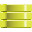This site uses cookies. By continuing to browse the ConceptDraw site you are agreeing to our Use of Site Cookies.

# Network Diagram Examples

ConceptDraw DIAGRAM diagramming software includes huge collection of network diagrams examples, computer and network templates, design objects and stencils.##Entity-Relationship Diagram (ERD)

Entity-Relationship Diagram (ERD) solution extends ConceptDraw DIAGRAM software with templates, samples and libraries of vector stencils from drawing the ER-diagrams by Chen's and crow’s foot notations.

## Network Diagram Examples

The vector stencils library "Sentence diagrams" contains 18 shapes for drawing sentence diagrams or parse trees.
"In pedagogy and theoretical syntax, a sentence diagram or parse tree is a pictorial representation of the grammatical structure of a sentence. The term "sentence diagram" is used more in pedagogy, where sentences are diagrammed. The term "parse tree" is used in linguistics (especially computational linguistics), where sentences are parsed. The purpose of sentence diagrams and parse trees is to have a model of the structure of sentences. The model is informative about the relations between words and the nature of syntactic structure and is thus used as a tool to help predict which sentences are and are not possible." [Sentence diagram. Wikipedia]
The shapes example "Design elements - Sentence diagrams" was created using the ConceptDraw PRO diagramming and vector drawing software extended with the Language Learning solution from the Science and Education area of ConceptDraw Solution Park.
Used Solutions